﻿ Notebook, Formulas, Mathematical Graphics, Homework, Mobile Phone Stock Photo - Image of lesson, interesting: 154138772

Notebook, formulas, mathematical graphics, homework, mobile phone.

Notebook, formulas, mathematical graphics, homework mobile phone pen pencil

More similar stock images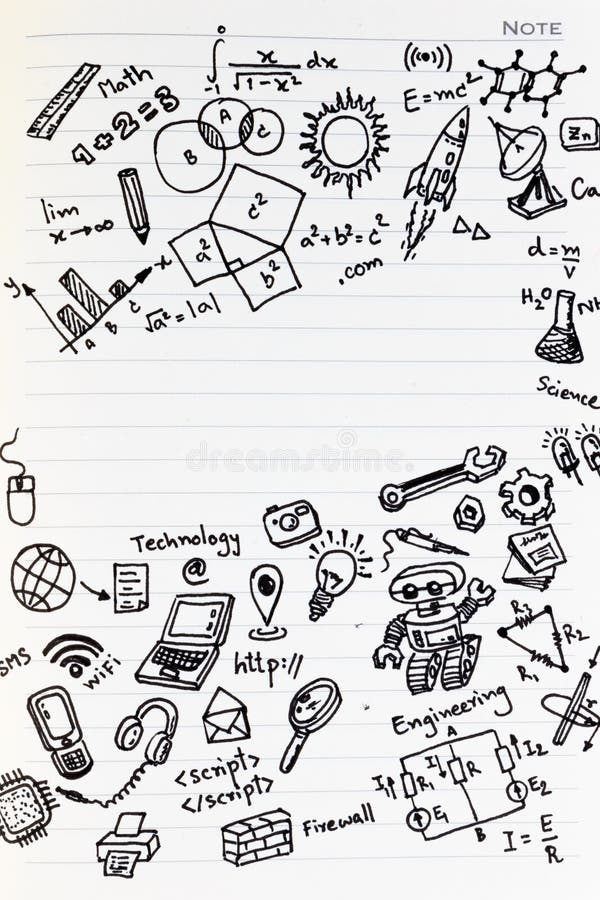Math formulas on notebook with copy space. Studying math back to school.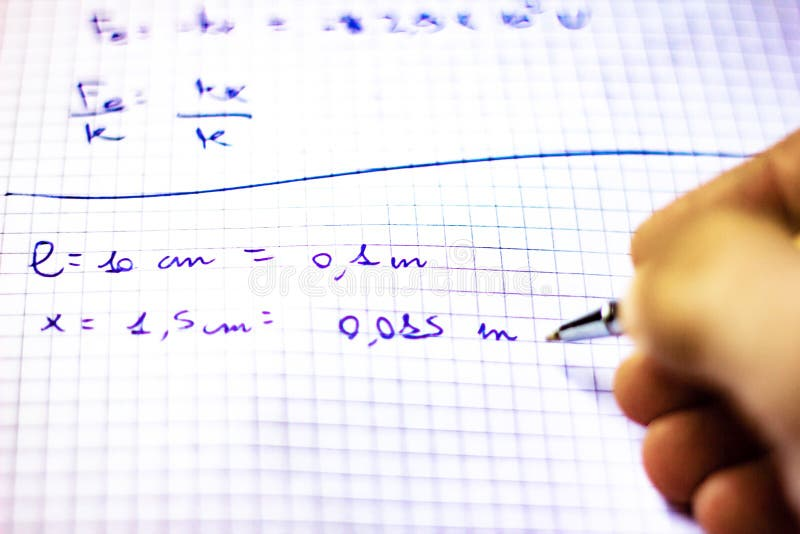Pen on a notebook with squares written with formulas, school starts in September, now it is just a short while away.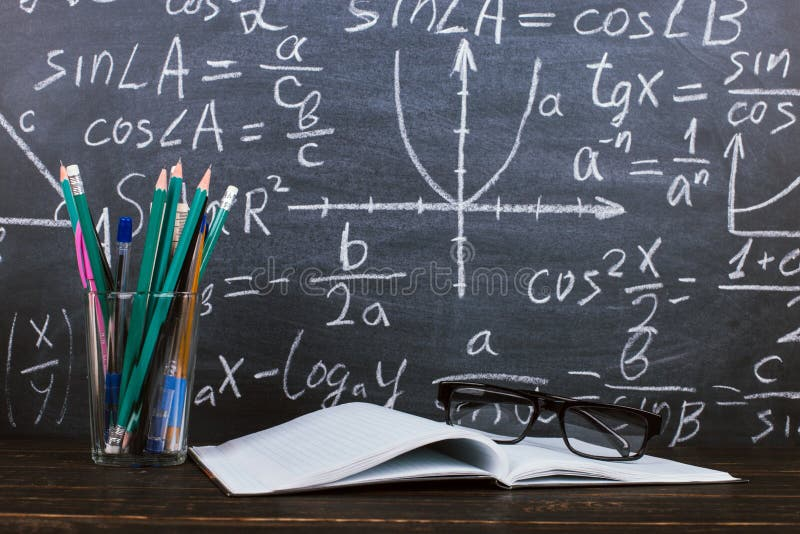Notebook, glasses and a glass with pencils on chalkboard background with formulas. Teacher's day concept and back to school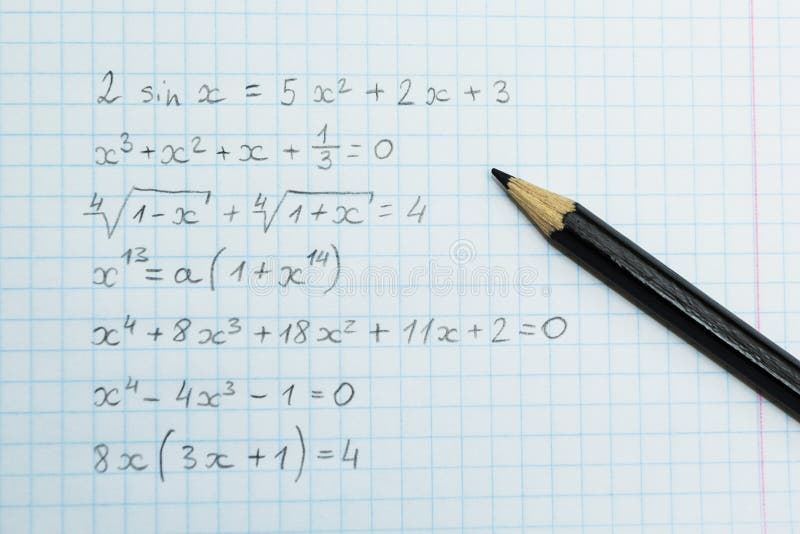Homework on arithmetic. Formulas in the notebook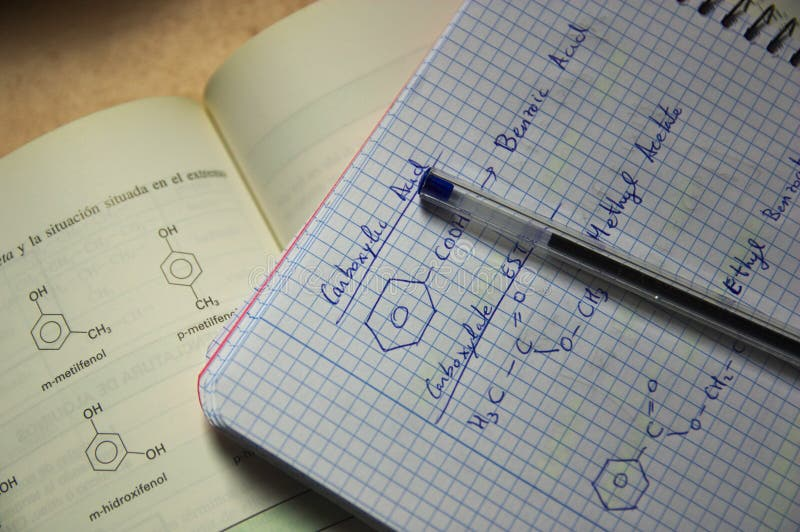A notebook and a book with formulas of organic chemistryTitle Back to school, formulas written by white chalk on the black school chalkboard and notebook with pencil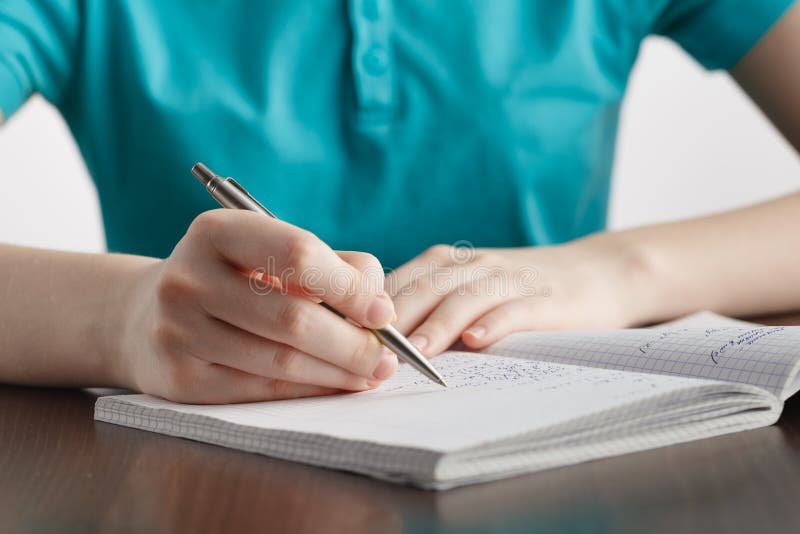Girl writes in a notebook mathematical formulas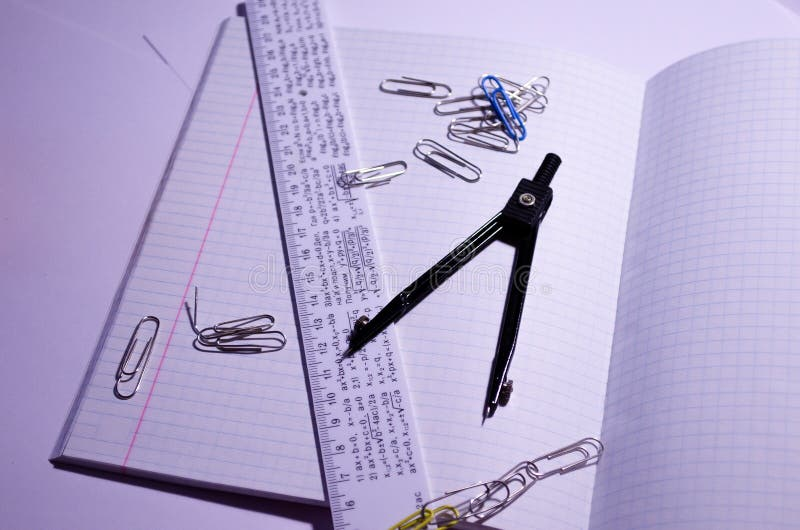An open notebook with paper clips, a ruler and a compass. Open notebook in a cage with school accessories. Office Chancellery.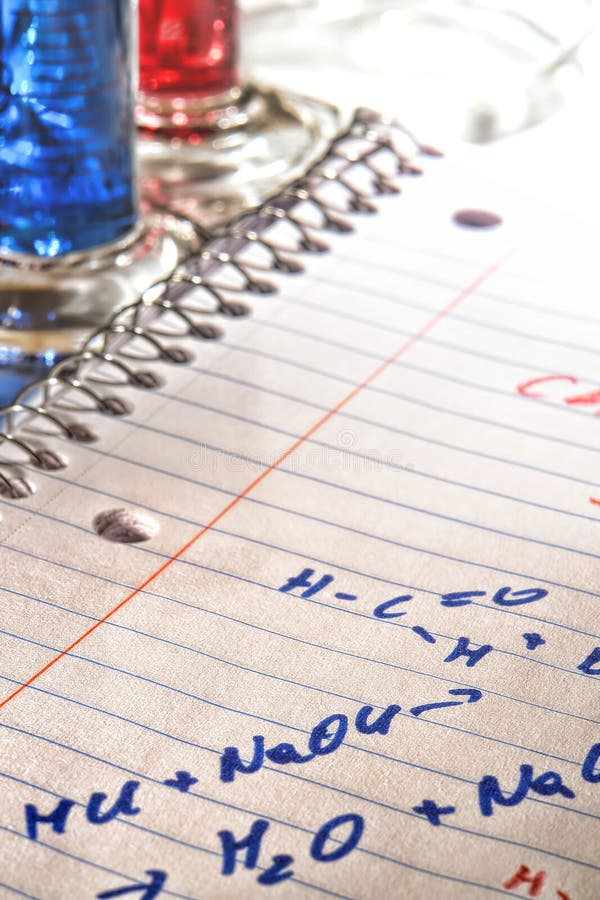Chemistry Formulas in Science Research Lab
Related categories